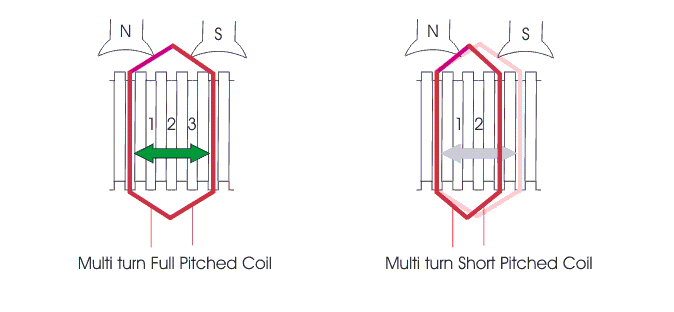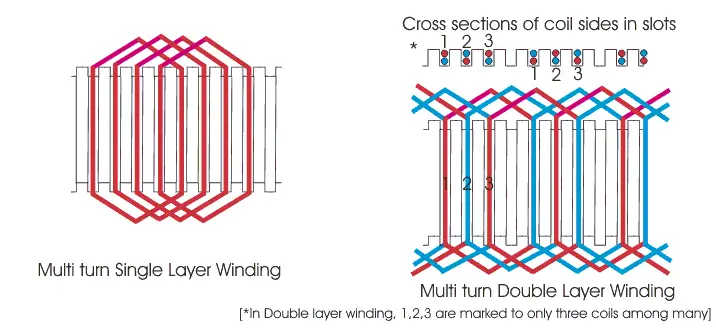# Armature Windings: Pole Pitch, Coil Span And Commutator Pitch

Contents

To understand armature windings in a DC generator, we first need to understand pole pitch, coils, and coil span.

## What is Pole Pitch?

Pole pitch is defined as the peripheral distance between the center of two adjacent poles in a DC machine. This distance is measured in terms of armature slots or armature conductors that come between two adjacent pole centers.

Pole Pitch is equal to the total number of armature slots divided by the total number of poles in the machine.

For example, if there are 96 slots on the armature periphery and 4 poles, the number of armature slots that come between two adjacent pole centers would be 96/4 = 24. Hence, the pole pitch of that DC machine would be 24.

Hence pole pitch is equal to total numbers of armature slots divided by total numbers of poles, we alternatively refer to it as armature slots per pole.

The formula for pole pitch and a worked example is discussed in the video below:

## What is a Coil?

The coil of the DC machine is made up of one turn or multi turns of the conductor. If the coil is made up of a single turn or a single loop of the conductor, it is called a single turn coil. If the coil is made up of more than one turn of a conductor, we refer to it as a multi-turn coil.

A single turn coil will have one conductor per side of the coil whereas, in multi turns coil, there will be multiple conductors per side of the coil. Whatever may be the number of conductors per side of the coil, each coil side is placed inside one armature slot only.

That means all conductors of one side of a particular coil must be placed in one single slot only. Similarly, we place all conductors on the opposite side of the coil in another single armature slot.

Coils and coil span is discussed in more detail in the video below.

## What is Coil Span (Coil Pitch)?

Coil span (also known as coil pitch) is defined as the peripheral distance between two sides of a coil, measured in terms of the number of armature slots between them. That means, after placing one side of the coil in a particular slot, after how many conjugative slots, the other side of the same coil is placed on the armature. This number is known as coil span.If the coil span is equal to the pole pitch, then the armature winding is said to be full – pitched. In this situation, two opposite sides of the coil lie under two opposite poles.

Hence emf induced in one side of the coil will be in a 180o phase shift with emf induced in the other side of the coil. Thus, the total terminal voltage of the coil will be nothing but the direct arithmetic sum of these two EMFs.

If the coil span is less than the pole pitch, then the winding is referred to as fractional pitched. In this coil, there will be a phase difference between induced EMFs on two sides, less than 180o. Hence resultant terminal voltage of the coil is the vector sum of these two EMFs and it is less than that of the full-pitched coil.In practice, coil pitch (or Span) as low as eight-tenths of a Pole Pitch, is employed without much serious reduction in emf. Fractional pitched windings are purposely used to effect a substantial saving in copper of the end connection and for improving commutation.

## Pitch of Armature Winding

### Back Pitch (Yb)

A coil advances on the back of the armature. This advancement is measured in terms of armature conductors and is called back pitch. It is equal to the number difference of the conductor connected to a given segment of the commutator.

### Front Pitch (Yf)

The number of armature conductors or elements spanned by a coil on the front is called front pitch.

Alternatively, we define the front-pitch as the distance between the second conductor of the next coil which connects the front, i.e., commutator end of the armature.

In other words, it is the number difference of the conductors connected together at the back end of the armature. We are showing both front and back pitches for a lap, and wave windings in the figure below.

### Resultant Pitch (Y)

It is the distance between the beginning of one coil and the beginning of the next coil to which it is connected.

As a matter of precautions, we should keep in mind that all these pitches, though normally stated concerning armature conductors, are also times of armature slots or commutator bars.

### Commutator Pitch

Commutator pitch is defined as the distance between two commutator segments in which two ends of the same armature coil are connected. We measure commutator pitch in terms of commutator bars or segments.

### Single Layer Armature Winding

We place armature coil sides in the armature slots differently. In some arrangement, each one side of an armature coil occupies a single slot.

In other words, we place one coil side in each armature slot. We refer to this arrangement as single-layer winding.### Two Layer Armature Winding

In other types of armature winding, arrangement two coil sides occupy every armature slot; one occupies the upper half, and another one occupies the lower half of the slot.

We so place the coils in two layers winding that if one side occupies upper half, then another side occupies the lower half of some other slot at a distance of one coil pitch away.

Want To Learn Faster? 🎓
Get electrical articles delivered to your inbox every week.
No credit card required—it’s 100% free.